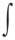GLOBAL WARMING & AEROSOLS
Clifford E Carnicom
Feb 23 2004

FURTHER DISCUSSION

The fundamental equations that address the heating of the atmosphere with the introduction of foreign materials are the following:

cv = sum [mfi * cvi]

which is the specific heat of a mixture (gravimetric analysis)2

where

mfi is the mass fraction of the ith component, and cvi is the specific heat of the ith component in units of joules / (kg * oK)

and cv is the specific heat of the mixture in units of joules / (kg * oK) and oK is degrees Kelvin.

and the heat transfer as given by the first law of thermodynamics3

Q = m * cv * del T

where Q represents the change in energy in joules, m is the total mass of the mixture, and del T is the change in degrees of the mass in degrees Kelvin.

Let us assume the atmosphere as a shell around the earth of variable height, the volume of which is given by:

vair = ( 4 / 3) * pi * [ ( R + upper )3 – ( R + lower)3 ]

where vair is the volume of the atmospheric shell in cubic meters, R is the mean radius of the earth in meters, upper is the upper limit of the atmospheric shell under consideration in meters (above sea level), and lower is the lower limit of the atmospheric shell in meters (above sea level).

Based upon an exponential regression of atmospheric density data in kilograms4, a suitable model for the mass of a column of air 1 meter square in dimension can be developed in the following form:

mair =1.474 * exp -1.424E-4 * h dh

integrated with respect to the upper and lower limits of the atmospheric shell, and mair is the mass of the atmospheric shell in kilograms, and h is in meters.

The mass of the aerosol in kilograms within an atmospheric column of air 1 meter square in dimension is expressed as:

ma = da * (upper – lower)

where the density of a particular aerosol in units of kilograms is designated as da.

As the density of the aerosol and the atmosphere will be considered to be uniform throughout the shell considered, the mass fractions of the atmosphere and the aerosol contribution, respectively, are:

mfair = mair / (mair + ma)

and

mfa = ma / (mair + ma)

Therefore:

cv = ( mfa * cva ) + (mfair * cvair)

where cva and cvair are the constant volume specific heats of the aerosol and air, respectively.

since Q = m * cv * del T

and since we are interested in the change in Q that results from a change in the specific heat of the mixture, we have:

dQ = matotal* del T * dcv

where dQ represents the change in energy in joules that results from a change of temperature in the atmospheric shell in degrees Kelvin and a change in the specific heat of the atmosphere from the introduction of an aerosol component within this mixture. The total mass of the atmospheric shell is given by matotal.

where matotal = mair * vair

and dcv = cv – cvair

It will be found that all introduced materials with a specific heat of less than 1003 joules / (kg * oK) (the specific heat of air) will lead to a decrease in the amount of energy required to raise the temperature of the mass of the atmospheric shell by 1 degree Kelvin.  Since the energy from the sun can be considered as a relative constant for the problem of concern, this solar energy will result in an increase in the temperature of the atmospheric shell.  The specific heat of barium, for example is approximately 190 joules / (kg * oK).5 This particular element will have highly significant thermodynamic impacts upon the lower atmosphere; the effect of the vast majority of metals and most chemical elements is significant as well.

1. Clifford E Carnicom, Drought Inducement, (http://carnicominstitute.org/wp/drought-inducement/), 04/07/02
2. Merle C. Potter, Thermodynamics for Engineers, (McGraw Hill, 1993), 251.
3. Potter, 251.
4. David R. Lide, CRC Handbook of Chemistry and Physics, (CRC Press, 2001), 14-19 to 14-22.
5. Carnicom, 04/07/02.